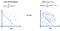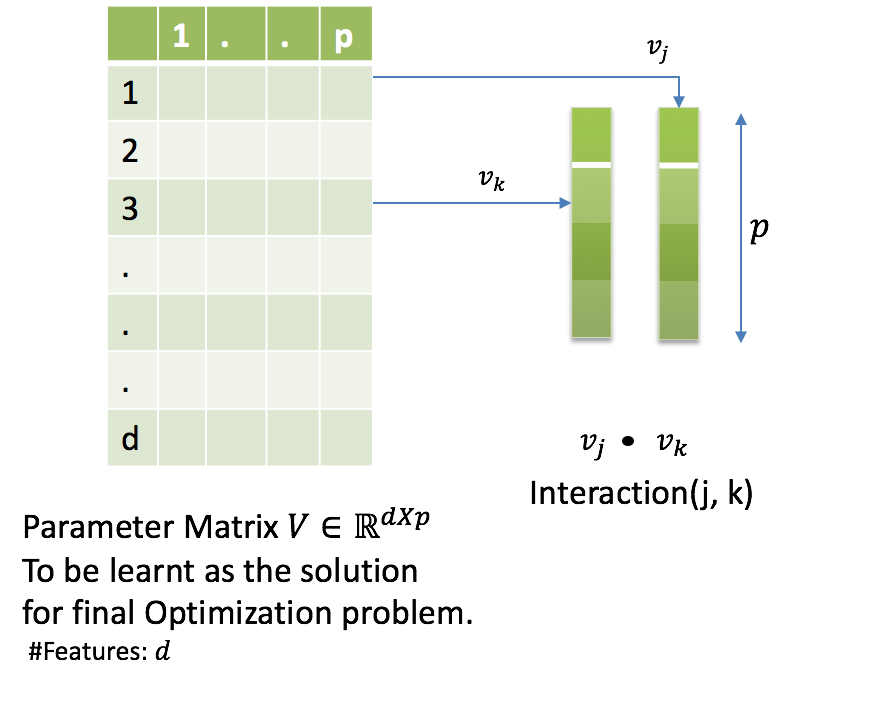# Robust Factorization Machines

Incomplete data? Noisy settings? Lets explore the Robust Factorization Machines, a recent noise-proof addition in the supervised learning domain.

Robust Factorization Machines, proposed recently at the WWW’18, are a family of non-linear classifiers that take into account any potential data incompleteness/noise. They incorporate the principles of Robust Optimization in the highly expressive Factorization machines. As a result the trained models exhibit high noise resilience.

This blog attempts to provide an intuitive understanding about the Robust Factorization Machines. We will skip most of the maths and proofs and such.

Please refer the original paper for a rigorous mathematical explanation. This blog beautifully captures the motivations behind the paper and how robustness is desirable in user response prediction domain.

1. Robustness,
2. Factorization Machines.

# Robustness

How does the most basic Machine Learning (ML) Pipeline look like?
Take some ML classifier, feed data into it and out comes a model! Simple.

Data quality matters a lot! Data Scientists spend a large amount of time working on getting a ‘clean dataset’. But as many would agree, there is only so much data engineering and cleaning that can be done.

What if a classifier could take care of it ❤?

## Robust Classifiers over Standard Classifiers?

The classifiers differ in their treatment of the training data and consequently how the optimization problems are framed.

Standard classifiers:
- assume data is precisely known.
- framed as a Loss-minimization problem w.r.t. a weight vector (w) being learnt
- represented in Fig. 1 (a).

Robust classifiers:
- assume uncertainty associated with each data point. Notion of deterministic, set based uncertainty U. See Eq. 1. This allows for the data point to now exist anywhere in a hyper-rectangular manifold. See Fig. 1.
- framed as a Minimax problem, minimizing loss w.r.t. a weight vector (w) while also maximizing w.r.t. an uncertainty (U).
- represented in Fig. 1 (b).Eq. 1. Uncertainty set definition. Uncertainty is defined over each datapoint. Here x represents a single data point and m is the number of data points.Fig. 1. (a) Standard classifier v/s (b) Robust classifier. Note how the introduction of uncertainty in (b) results in ‘hyper-rectangles’ over the data points, thus leading to change in the learnt classifier boundary.

In a nutshell, Robust Optimization seeks to learn a classifier that remains feasible and near optimal under worst case uncertainty realization.

# Factorization Machines

Lets take a classification scenario.
For a purchase prediction problem with two features (item_category and device), if we know ‘clothing’ category is frequently purchased on ‘mobiles’ and not on ‘desktop’, how do we capture such feature interactions? How does a model capture the importance that a feature interaction such as ‘device=mobile and category=clothing’ has over considering only the individual features? A linear model alone does not suffice.

Factorization Machines(FMs), proposed by Steffen Rendle, are a family of non-linear classifiers designed to capture feature-interactions in a latent space. That is, for every feature a p-dimensional vector is learnt, resulting in a d x p dimensional weight matrix, where d is original no. of features. The similarity between two features is then given by the dot product of these latent vectors e.g. as in Fig 2, interaction strength b/w features j and k will be computed as a dot product over the following two vectors: latent vector for feature j (v_j) and latent vector for feature k (v_k).Fig. 2. Computation of similarity b/w feature j and feature k. Parameter Matrix V is learnt s.t. similarity of features j and k is computed using a dot product b/w rows j and k of the matrix V.

# Robust Factorization Machines

Now that we have developed some intuition about robustness and factorization machines, its time to uncover key aspects of the paper titled “Robust Factorization Machines for User Response Prediction”.

The paper is motivated using the noisy and incomplete data available in the User Response Prediction problem. Checkout our blog describing the need for robustness in the domain.

The key idea is to extend Factorization machines using principles of Robust optimization. The resulting Minimax formulation is then converted to pure minimization problem by deriving upper bounds on loss w.r.t. an uncertainty matrix U.

The paper proposes two novel algorithms:
- Robust Factorization Machines (RFM).
- Robust Field Aware Factorization Machines (RFFM).

Extensive experiments on real-world large-scale datasets give insights into the performance and scalability of the proposed algorithms.

Promising results:
- Significant reduction (4.45% to 38.65%) in Logloss in Noisy settings.
- Slight performance hit (-0.24% to -1.1%) in Noise-free setting.

An open-source Spark-based distributed implementation for the proposed algorithms is available here. Evaluating RFMs and RFFMs across a breadth of classification scenarios is an interesting area to explore.

RFMs and RFFMs are domain-independent formulations, applicable in any domain with noisy/incomplete data.

## Bringing robustness to the forefront

With the increasing noise in the input signals, it is important to design classifiers which embrace this uncertainty. RFMs and RFFMs are a step in this direction. Incorporating robustness in tree ensembles and deep neural networks is a promising area of investigation.

## Walmart Global Tech Blog

We’re powering the next great retail disruption.

Written by

## Priyanka Bhatt

Senior Manager, Data Science @WalmartLabs, Bangalore | IISc, Bangalore